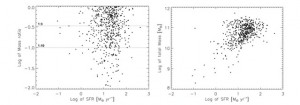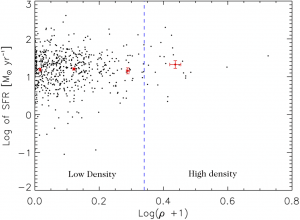The infrared properties of mergers

Another update from Alfredo Carpineti:

Following the previous post, we continue the analysis of galaxy mergers in the infrared.

We want to understand where our galaxies stand with respect to other mergers and other infrared luminous galaxies. Using infrared radiation we can extrapolate the number of stars produced by a galaxy every year, namely the star formation rate(SFR). This number is really important since the star content of a galaxy modifies  both its colours and its intrinsic properties. The average star formation rate is around 15 solar masses per year, which is high, considered that the SFR for a common galaxy is of 1-2 solar masses per year.Let’s compare now the SFR with the mass. We can use two parameters to define the mass of a merger: the total mass and the mass ratio. The total mass is the sum of the masses of the two galaxies while the mass ratio is the ratio between the two masses. If a merger had a mass ratio between  1:1 and 1:3 is called a major merger, otherwise it’s a minor merger.
From the plot you can see that we don’t find any correlation between SFR and mass ratio, while we see a clear trend with the total mass.Another interesting parameter is the environment density. Density variations give way to difference in the tidal forces, approaching velocities and concentration of intergalactic gas and dust. These could lead to a dependence of the SFR on the environment. When we looked for it we found no such thing. The star formation rate seems independent of environment.